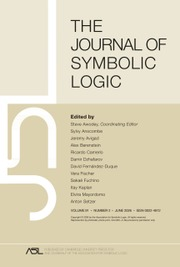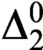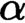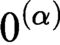Home
Hostname: page-component-684899dbb8-t7hbd Total loading time: 0.335 Render date: 2022-05-17T19:08:38.405Z Has data issue: true Feature Flags: { "shouldUseShareProductTool": true, "shouldUseHypothesis": true, "isUnsiloEnabled": true, "useRatesEcommerce": false, "useNewApi": true }The Journal of Symbolic Logic

# COMPUTABILITY OF POLISH SPACES UP TO HOMEOMORPHISM

Published online by Cambridge University Press:  26 October 2020

## Abstract

We study computable Polish spaces and Polish groups up to homeomorphism. We prove a natural effective analogy of Stone duality, and we also develop an effective definability technique which works up to homeomorphism. As an application, we show that there is a$\Delta ^0_2$ Polish space not homeomorphic to a computable one. We apply our techniques to build, for any computable ordinal$\alpha$, an effectively closed set not homeomorphic to any$0^{(\alpha )}$-computable Polish space; this answers a question of Nies. We also prove analogous results for compact Polish groups and locally path-connected spaces.

## MSC classification

Type
Articles
Information
The Journal of Symbolic Logic , December 2020 , pp. 1664 - 1686
© The Association for Symbolic Logic 2020

## Access options

Get access to the full version of this content by using one of the access options below. (Log in options will check for institutional or personal access. Content may require purchase if you do not have access.)

## References

Ash, C., Recursive labeling systems and stability of recursive structures in hyperarithmetical degrees. Transactions of the American Mathematical Society, vol. 298 (1986). pp. 497514.CrossRefGoogle Scholar
Ash, C. and Knight, J., Computable Structures and the Hyperarithmetical Hierarchy, Studies in Logic and the Foundations of Mathematics, vol. 144, North-Holland, Amsterdam, Netherlands, 2000.Google Scholar
Brattka, V. and Gherardi, G., Borel complexity of topological operations on computable metric spaces. Journal of Logic and Computation, vol. 19 (2009), no. 1, pp. 4576.CrossRefGoogle Scholar
Brattka, V., Hertling, P., and Weihrauch, K., A tutorial on computable analysis, New Computational Paradigms (Cooper, S. B., Löwe, B., and Sorbi, A., editors), Springer, New York, 2008, pp. 425491.CrossRefGoogle Scholar
Brown, T. A., Computable structure theory on banach spaces, Ph.D thesis, Iowa State University, ProQuest LLC, Ann Arbor, 2019.Google Scholar
Cenzer, D. A. and Remmel, J. B., Index sets in computable analysis. Theoretical Computer Science, vol. 219 (1999), no. 1-2, pp. 111150.CrossRefGoogle Scholar
Clanin, J., McNicholl, T. H., and Stull, D. M., Analytic computable structure theory and${L}^p$spaces. Fundamenta Mathematicae, vol. 244 (2019), no. 3, pp. 255285.CrossRefGoogle Scholar
Downey, R. and Jockusch, C. G., Every low Boolean algebra is isomorphic to a recursive one. Proceedings of the American Mathematical Society, vol. 122 (1994), no. 3, 871880.CrossRefGoogle Scholar
Downey, R. G. and Melnikov, A. G., Computable analysis and classification problems, Beyond the Horizon of Computability (Anselmo, M., Vedova, G. D., Manea, F., and Pauly, A., editors), Springer, Cham, 2020, pages 100111.CrossRefGoogle Scholar
Dobritsa, V., Some constructivizations of abelian groups. Siberian Journal of Mathematics, vol. 24 (1983), pp. 167173 (in Russian).CrossRefGoogle Scholar
Ershov, Y. and Goncharov, S., Constructive Models. Siberian School of Algebra and Logic, Consultants Bureau, New York, 2000.CrossRefGoogle Scholar
Feiner, L., Hierarchies of Boolean algebras, this Journal, vol. 35 (1970), pp. 365374.Google Scholar
Ge, X. and Richards, J. I., Computability in unitary representations of compact groups, Logical Methods (Ithaca, NY, 1992) (Crossley, J. N., Remmel, J. B., Shore, R. A., and Sweedler, M. E., editors), Progress in Computer Science and Applied Logic, vol. 12, Birkhäuser, Boston, 1993, pp. 386421.CrossRefGoogle Scholar
Goncharov, S. S., Countable Boolean Algebras and Decidability. Siberian School of Algebra and Logic, Consultants Bureau, New York, 1997.Google Scholar
Goncharov, S. and Knight, J., Computable structure and antistructure theorems. Algebra Logika, vol. 41 (2002), no. 6, pp. 639681, 757.Google Scholar
Greenberg, N., Melnikov, A. G., Knight, J. F., and Turetsky, D., Uniform procedures in uncountable structures, this Journal, vol. 83 (2018), no. 2, pp. 529550.Google Scholar
Greenberg, N., Melnikov, A., Nies, A., and Turetsky, D., Effectively closed subgroups of the infinite symmetric group. Proceedings of the American Mathematical Society, vol. 146 (2018), no. 12, pp. 54215435.CrossRefGoogle Scholar
Greenberg, N. and Montalbán, A., Ranked structures and arithmetic transfinite recursion. Transactions of the American Mathematical Society, vol. 360 (2008), no. 3, pp. 12651307.CrossRefGoogle Scholar
Grubba, T. and Weihrauch, K., On computable metrization. Electronic Notes in Theoretical Computer Science, vol. 167 (2007), pp. 345364.CrossRefGoogle Scholar
Hoyrup, M., Kihara, T., and Selivanov, V., Degree spectra of homeomorphism types of Polish spaces. Preprint, 2020. Available at https://hal.inria.fr/hal-02555111 and arXiv:2004.06872.CrossRefGoogle Scholar
Hoyrup, M., Rojas, C., Selivanov, V. L., and Stull, D. M., Computability on quasi-polish spaces, Descriptional Complexity of Formal Systems - 21st IFIP WG 1.02 International Conference, DCFS 2019, Košice, Slovakia, July 17-19, 2019 (Hospodár, M., Jirásková, G., and Konstantinidis, S., editors), Lecture Notes in Computer Science, vol. 11612, Springer, New York, 2019, pp. 171183.Google Scholar
Jarden, M., Algebraic extensions of finite corank of Hilbertian fields. Israel Journal of Mathematics, vol. 18 (1974), pp. 279307.CrossRefGoogle Scholar
Jockusch, C. G. and McLaughlin, T. G., Countable retracing functions and predicates. Pacific Journal of Mathematics, vol. 30 (1969), no. 1, pp. 6793.CrossRefGoogle Scholar
Khisamiev, N., Constructive Abelian groups, Handbook of Recursive Mathematics (Ershov, Yu. L., Goncharov, S.S., Nerode, A., Remmel, J.B., and Marek, V.W., editors), North-Holland, Amsterdam, 1998, pp. 11771231.Google Scholar
Knight, J. F. and Stob, M., Computable Boolean algebras, this Journal, vol. 65 (2000), no. 4, pp. 16051623, 12.Google Scholar
Roche, P. L., Effective Galois theory, this Journal, vol. 46 (1981), no. 2, pp. 385392.Google Scholar
McNicholl, T. H., Computable copies of lp. Computability, vol. 6 (2017), no. 4, pp. 391408.CrossRefGoogle Scholar
Melnikov, A. G., Computably isometric spaces, this Journal, vol. 78 (2013), no. 4, pp. 10551085.Google Scholar
Melnikov, A. G., Computable Abelian groups. The Bulletin of Symbolic Logic, vol. 20 (2014), no. 3, pp. 315356.CrossRefGoogle Scholar
Melnikov, A. G., Computable topological groups and Pontryagin duality. Transactions of the American Mathematical Society, vol. 370 (2018), no. 12, pp. 87098737.CrossRefGoogle Scholar
Melnikov, A. and Montalbán, A., Com putable Polish group actions, this Journal, vol. 83 (2018), no. 2, pp. 443460.Google Scholar
Melnikov, A. G. and Ng, K. M., Computable structures and operations on the space of continuous functions. Fundamenta Mathematicae, vol. 233 (2016), no. 2, pp. 101141.Google Scholar
Melnikov, A. G. and Nies, A., The classification problem for compact computable metric spaces, The Nature of Computation (Bonizzoni, P., Brattka, V., and Löwe, B., editors), Lecture Notes in Computer Science, vol. 7921, Springer, Heidelberg, 2013, pp. 320328.Google Scholar
McNicholl, T. H. and Stull, D. M.. The isometry degree of a computable copy of${\ell}^p$. Computability, vol. 8 (2019), no. 2, pp. 179189. Available online at https://content.iospress.com/articles/computability/com180214.CrossRefGoogle Scholar
Myhill, J., A recursive function, defined on a compact interval and having a continuous derivative that is not recursive. Michigan Mathematical Journal, vol. 18 (1971), pp. 9798.CrossRefGoogle Scholar
Nies, A. and Solecki, S., Local compactness for computable polish metric spaces is${\varPi}_1^1$-complete. Evolving Computability - 11th Conference on Computability in Europe, 2015, pp. 286–290.CrossRefGoogle Scholar
Pour-El, M. B. and Richards, I., Computability and noncomputability in classical analysis. Transactions of the American Mathematical Society, vol. 275 (1983), no. 2, pp. 539560.CrossRefGoogle Scholar
Pour-El, M. B. and Richards, I., Computability in Analysis and Physics, Perspectives in Mathematical Logic, Springer-Verlag, Berlin, 1989.CrossRefGoogle Scholar
Pontryagin, L. S., Topological Groups, Translated from the second Russian edition by Arlen Brown. Gordon and Breach Science, New York-London-Paris, 1966.Google Scholar
Sacks, G. E., Higher Recursion Theory, Perspectives in Mathematical Logic, Springer-Verlag, Berlin, 1990.CrossRefGoogle Scholar
Selivanov, V. L., On degree spectra of topological spaces. Lobachevskii Journal of Mathematics, vol. 41 (2020), pp. 252259.CrossRefGoogle Scholar
Smith, R. L., The theory of profinite groups with effective presentations, Ph.D. thesis, The Pennsylvania State University, ProQuest LLC, Ann Arbor, 1979.Google Scholar
Smith, R. L., Effective aspects of profinite groups, this Journal, vol. 46 (1981), no. 4, pp. 851863.Google Scholar
Turing, A. M., On computable numbers, with an application to the Entscheidungsproblem. Proceedings of the London Mathematical Society, vol. 42 (1936), pp. 230265.Google Scholar
Turing, A. M., On computable numbers, with an application to the Entscheidungsproblem. A correction. Proceedings of the London Mathematical Society, vol. 43 (1937), pp. 544546.Google Scholar
Weihrauch, K., Computable Analysis, Texts in Theoretical Computer Science, Springer-Verlag, Berlin, 2000.CrossRefGoogle Scholar
Weihrauch, K. and Grubba, T., Elementary computable topology. Journal of Universal Computer Science, vol. 15 (2009), no. 6, pp. 13811422.Google Scholar
Yasugi, M., Mori, T., and Tsujii, Y., Effective properties of sets and functions in metric spaces with computability structure. Theoretical Computer Science, vol. 219 (1999), no. 1-2, pp. 467486.CrossRefGoogle Scholar

# Save article to Kindle

Note you can select to save to either the @free.kindle.com or @kindle.com variations. ‘@free.kindle.com’ emails are free but can only be saved to your device when it is connected to wi-fi. ‘@kindle.com’ emails can be delivered even when you are not connected to wi-fi, but note that service fees apply.

Find out more about the Kindle Personal Document Service.

COMPUTABILITY OF POLISH SPACES UP TO HOMEOMORPHISM
Available formats
×

# Save article to Dropbox

To save this article to your Dropbox account, please select one or more formats and confirm that you agree to abide by our usage policies. If this is the first time you used this feature, you will be asked to authorise Cambridge Core to connect with your Dropbox account. Find out more about saving content to Dropbox.

COMPUTABILITY OF POLISH SPACES UP TO HOMEOMORPHISM
Available formats
×

# Save article to Google Drive

To save this article to your Google Drive account, please select one or more formats and confirm that you agree to abide by our usage policies. If this is the first time you used this feature, you will be asked to authorise Cambridge Core to connect with your Google Drive account. Find out more about saving content to Google Drive.

COMPUTABILITY OF POLISH SPACES UP TO HOMEOMORPHISM
Available formats
×
×